## Programming Assignment 1: Percolation

Write a program to estimate the value of the percolation threshold via Monte Carlo simulation.

Install a Java programming environment. Install a Java programming environment on your computer by following these step-by-step instructions for your operating system [ Mac OS X · Windows · Linux ]. After following these instructions you will have stdlib.jar and algs4.jar in your Java classpath: the former contains libraries for reading data from standard input, writing data to standard output, drawing results to standard draw, generating random numbers, computing statistics, and timing programs; the latter contains all of the algorithms in the textbook.

Percolation. Given a composite systems comprised of randomly distributed insulating and metallic materials: what fraction of the materials need to be metallic so that the composite system is an electrical conductor? Given a porous landscape with water on the surface (or oil below), under what conditions will the water be able to drain through to the bottom (or the oil to gush through to the surface)? Scientists have defined an abstract process known as percolation to model such situations.

The model. We model a percolation system using an N-by-N grid of sites. Each site is either open or blocked. A full site is an open site that can be connected to an open site in the top row via a chain of neighboring (left, right, up, down) open sites. We say the system percolates if there is a full site in the bottom row. In other words, a system percolates if we fill all open sites connected to the top row and that process fills some open site on the bottom row. (For the insulating/metallic materials example, the open sites correspond to metallic materials, so that a system that percolates has a metallic path from top to bottom, with full sites conducting. For the porous substance example, the open sites correspond to empty space through which water might flow, so that a system that percolates lets water fill open sites, flowing from top to bottom.)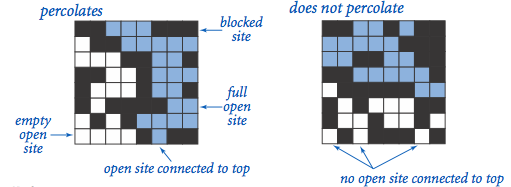The problem. In a famous scientific problem, researchers are interested in the following question: if sites are independently set to be open with probability p (and therefore blocked with probability 1 − p), what is the probability that the system percolates? When p equals 0, the system does not percolate; when p equals 1, the system percolates. The plots below show the site vacancy probability p versus the percolation probability for 20-by-20 random grid (left) and 100-by-100 random grid (right).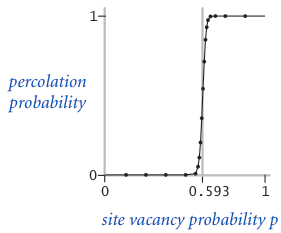When N is sufficiently large, there is a threshold value p* such that when p < p* a random N-by-N grid almost never percolates, and when p > p*, a random N-by-N grid almost always percolates. No mathematical solution for determining the percolation threshold p* has yet been derived. Your task is to write a computer program to estimate p*.

Percolation data type. To model a percolation system, create a data type Percolation with the following API:

```public class Percolation {
public Percolation(int N)               // create N-by-N grid, with all sites blocked
public void open(int i, int j)          // open site (row i, column j) if it is not open already
public boolean isOpen(int i, int j)     // is site (row i, column j) open?
public boolean isFull(int i, int j)     // is site (row i, column j) full?
public boolean percolates()             // does the system percolate?
}
```

Corner cases.  By convention, the row and column indices i and j are integers between 0 and N − 1, where (0, 0) is the upper-left site: Throw a java.lang.IndexOutOfBoundsException if any argument to open(), isOpen(), or isFull() is outside its prescribed range. The constructor should throw a java.lang.IllegalArgumentException if N ≤ 0.

Performance requirements.  The constructor should take time proportional to N2; all methods should take constant time plus a constant number of calls to the union-find methods union(), find(), connected(), and count().

Monte Carlo simulation. To estimate the percolation threshold, consider the following computational experiment:

• Initialize all sites to be blocked.

• Repeat the following until the system percolates:

• Choose a site (row i, column j) uniformly at random among all blocked sites.

• Open the site (row i, column j).

• The fraction of sites that are opened when the system percolates provides an estimate of the percolation threshold.

For example, if sites are opened in a 20-by-20 grid according to the snapshots below, then our estimate of the percolation threshold is 204/400 = 0.51 because the system percolates when the 204th site is opened.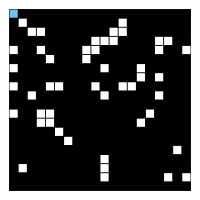50 open sites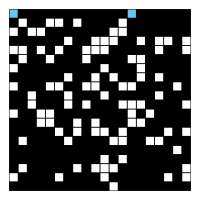100 open sites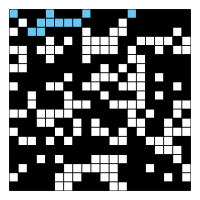150 open sites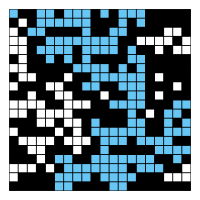204 open sites

By repeating this computation experiment T times and averaging the results, we obtain a more accurate estimate of the percolation threshold. Let xt be the fraction of open sites in computational experiment t. The sample mean μ provides an estimate of the percolation threshold; the sample standard deviation σ measures the sharpness of the threshold.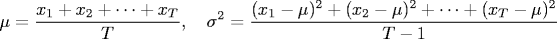Assuming T is sufficiently large (say, at least 30), the following provides a 95% confidence interval for the percolation threshold:
To perform a series of computational experiments, create a data type PercolationStats with the following API.

```public class PercolationStats {
public PercolationStats(int N, int T)   // perform T independent experiments on an N-by-N grid
public double mean()                    // sample mean of percolation threshold
public double stddev()                  // sample standard deviation of percolation threshold
public double confidenceLow()           // low  endpoint of 95% confidence interval
public double confidenceHigh()          // high endpoint of 95% confidence interval
}
```
The constructor should throw a java.lang.IllegalArgumentException if either N ≤ 0 or T ≤ 0.

The constructor should take two arguments N and T, and perform T independent computational experiments (discussed above) on an N-by-N grid. Using this experimental data, it should calculate the mean, standard deviation, and the 95% confidence interval for the percolation threshold. Use standard random from stdlib.jar to generate random numbers; use standard statistics from stdlib.jar to compute the sample mean and standard deviation.

```Example values after creating PercolationStats(200, 100)
mean()                  = 0.5929934999999997
stddev()                = 0.00876990421552567
confidenceLow()         = 0.5912745987737567
confidenceHigh()        = 0.5947124012262428

Example values after creating PercolationStats(200, 100)
mean()                  = 0.592877
stddev()                = 0.009990523717073799
confidenceLow()         = 0.5909188573514536
confidenceHigh()        = 0.5948351426485464

Example values after creating PercolationStats(2, 100000)
mean()                  = 0.6669475
stddev()                = 0.11775205263262094
confidenceLow()         = 0.666217665216461
confidenceHigh()        = 0.6676773347835391
```

Analysis of running time and memory usage. Implement the Percolation data type using the quick-find data type QuickFindUF.java from algs4.jar.

• Use the stopwatch data type from stdlib.jar to measure the total running time of PercolationStats. How does doubling N affect the total running time? How does doubling T affect the total running time? Give a formula (using tilde notation) of the total running time on your computer (in seconds) as a single function of both N and T.

• Using the 64-bit memory-cost model from lecture and Section 1.4, give the total memory usage in bytes (using tilde notation) that a Percolation object uses to model an N-by-N percolation system. Count all memory that is used, including memory for the union-find data structure.
Now, implement the Percolation data type using the weighted quick-union data type WeightedQuickUnionUF.java from algs4.jar. Answer the same questions in the previous two bullets.

Please wait until after Tuesday's lecture (Analysis of Algorithms) to answer these questions.

Deliverables. Submit only Percolation.java (using the weighted quick-union algorithm as implemented in the WeightedQuickUnionUF class) and PercolationStats.java. We will supply stdlib.jar and WeightedQuickUnionUF.java. On this assignment, the only library functions you may call are those in java.lang, stdlib.jar, and WeightedQuickUnionUF.java. Also, submit a readme.txt file and answer all questions. You will need to read the COS 226 Collaboration Policy in order to answer the related questions in your readme file.

This assignment was developed by Bob Sedgewick and Kevin Wayne.